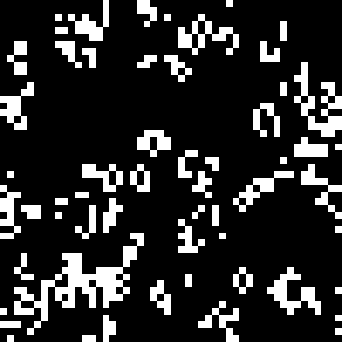Conway’s Game of Life has simple rules, but breeds unpredictable behaviour. The game proceeds on a regular grid as follows: you update all cells at once, cells survive if there are more than one, but less than four, cells in neighbouring sites. Dead cells are (re)born if they have exactly three neighbours. It was originally played with counters on a Go board, but rose to fame with the increasing availability of computers, which can play it faster. I won’t go into more detail because many excellent descriptions already exist:

Nevertheless, here are a couple of implementations which may be of interest.

## A Sedate Matlab Life

An exercise in conciseness, with the update rule complete in two statements and although it would be hard to beat a version in one line of APL, this Matlab code wins in the readability stakes! You’ll need Matlab; it runs with Octave too, but creates a new window for the grid at each timestep, so move the display outside the loop (and try `imshow` instead).

Update - someone emailed to tell me that Matlab actually plays life as a built-in function - just type life. But having looked at the code, while the method is obviously identical to the one below, I don’t mind claiming that the code here is a little more elegant.A stage of Life, as lived by Matlab
``````% life.m - Conway's Game of Life
%
% Cells are born to three adjacent parents,
% and die of overcrowding or loneliness.
%        Iain Haslam, December 2005

len=50; GRID=int8(rand(len,len));
up=[2:len 1]; down=[len 1:len-1]; %the world is round
colormap(gray(2));
for i=1:100
neighbours=GRID(up,:)+GRID(down,:)+GRID(:,up)+GRID(:,down)+...
GRID(up,up)+GRID(up,down)+GRID(down,up)+GRID(down,down);
GRID = neighbours==3 | GRID & neighbours==2;
image(GRID*2); pause(0.02);
end``````

The code as presented here starts with a random grid of living and dead cells. If you want to see a particular pattern, start with a blank grid GRID=zero(len,len), and add one of the following:

 Blinker GRID(5,4:6)=1; Toad GRID(5,4:6)=1;GRID(6,3:5)=1; Glider GRID(3,1:3)=1;GRID(2,3)=1;GRID(1,2)=1; Lightweight spaceship GRID(5,5:8)=1;GRID(4,9)=1;GRID(3:4,5)=1; GRID(2,6)=1;GRID(2,9)=1; Diehard GRID(10,10:11)=1;GRID(11,11)=1; GRID(11,15:17)=1;GRID(9,16)=1;

## Life in one long line of Matlab

It is of course possible to play the Game of Life in a single line of Matlab. It weighs in with twice the characters of the single line of APL (5^3 to be precise), but at least you can find them all on a normal keyboard. There will be scrolling.

``````l=9;G=int8(rand(l));u=[2:l 1];d=[l 1:l-1];for i=u,n=G(u,:)+G(d,:)+G(:,u)+G(:,d)+G(u,u)+G(u,d)+G(d,u)+G(d,d);G=n==3|G&n==2,end
``````